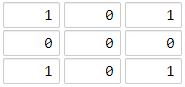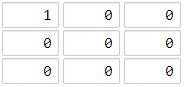# 1162. As Far from Land as Possible ⭐

Medium
Given an `n x n` `grid` containing only values `0` and `1`, where `0` represents water and `1` represents land, find a water cell such that its distance to the nearest land cell is maximized, and return the distance. If no land or water exists in the grid, return `-1`.
The distance used in this problem is the Manhattan distance: the distance between two cells `(x0, y0)` and `(x1, y1)` is `|x0 - x1| + |y0 - y1|`.
Example 1:Input: grid = [[1,0,1],[0,0,0],[1,0,1]]
Output: 2
Explanation: The cell (1, 1) is as far as possible from all the land with distance 2.
Example 2:Input: grid = [[1,0,0],[0,0,0],[0,0,0]]
Output: 4
Explanation: The cell (2, 2) is as far as possible from all the land with distance 4.
Constraints:
• `n == grid.length`
• `n == grid[i].length`
• `1 <= n <= 100`
• `grid[i][j]` is `0` or `1`

### 解題

func maxDistance(grid [][]int) int {
res := 0
for i:=0; i<len(grid); i++ {
for j:=0; j<len(grid); j++ {
if grid[i][j] == 1 { continue }
grid[i][j] = 201
if i > 0 {
grid[i][j] = min(grid[i][j], grid[i - 1][j] + 1)
}
if j > 0 {
grid[i][j] = min(grid[i][j], grid[i][j - 1] + 1)
}
}
}
for i:=len(grid)-1; i>=0;i-- {
for j:=len(grid)-1; j>=0; j-- {
if grid[i][j] == 1 { continue }
if i < len(grid) - 1 {
grid[i][j] = min(grid[i][j], grid[i + 1][j] + 1)
}
if j < len(grid) - 1 {
grid[i][j] = min(grid[i][j], grid[i][j + 1] + 1)
}
res = max(res, grid[i][j])
}
}
if res == 201 { return -1 }
return res - 1
}
func min(a, b int) int {
if a < b { return a }
return b
}
func max(a, b int) int {
if a > b { return a }
return b
}